Chemistry 2e

# Chapter 8

Chemistry 2eChapter 8

1.

Similarities: Both types of bonds result from overlap of atomic orbitals on adjacent atoms and contain a maximum of two electrons. Differences: σ bonds are stronger and result from end-to-end overlap and all single bonds are σ bonds; π bonds between the same two atoms are weaker because they result from side-by-side overlap, and multiple bonds contain one or more π bonds (in addition to a σ bond).

3.

The specific average bond distance is the distance with the lowest energy. At distances less than the bond distance, the positive charges on the two nuclei repel each other, and the overall energy increases.

5.

Bonding: One σ bond and one π bond. The s orbitals are filled and do not overlap. The p orbitals overlap along the axis to form a σ bond and side-by-side to form the π bond.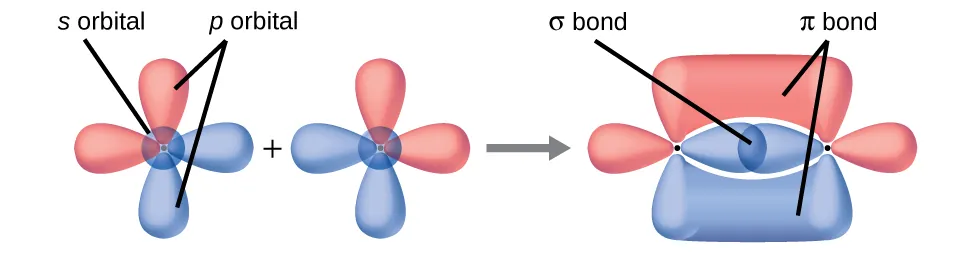7.

No, two of the p orbitals (one on each N) will be oriented end-to-end and will form a σ bond.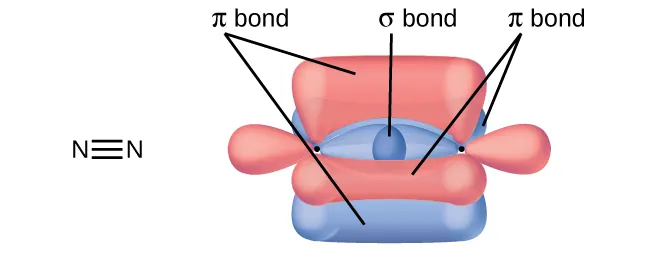9.

Hybridization is introduced to explain the geometry of bonding orbitals in valance bond theory.

11.

There are no d orbitals in the valence shell of carbon.

13.

trigonal planar, sp2; trigonal pyramidal (one lone pair on A) sp3; T-shaped (two lone pairs on A sp3d, or (three lone pairs on A) sp3d2

15.

(a) Each S has a bent (109°) geometry, sp3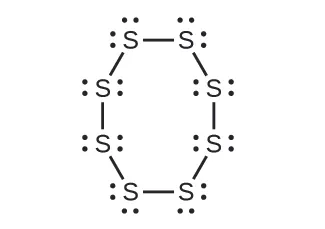(b) Bent (120°), sp2(c) Trigonal planar, sp2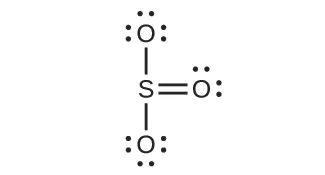(d) Tetrahedral, sp3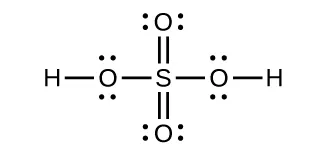17.

(a) XeF2
(b)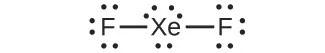(c) linear (d) sp3d

19.

(a)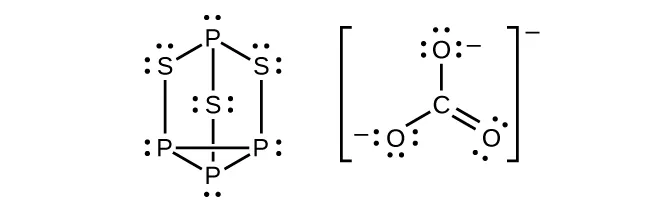(b) P atoms, trigonal pyramidal; S atoms, bent, with two lone pairs; Cl atoms, trigonal pyramidal; (c) Hybridization about P, S, and Cl is, in all cases, sp3; (d) Oxidation states P +1, S$−113,−113,$ Cl +5, O –2. Formal charges: P 0; S 0; Cl +2: O –1

21.Phosphorus and nitrogen can form sp3 hybrids to form three bonds and hold one lone pair in PF3 and NF3, respectively. However, nitrogen has no valence d orbitals, so it cannot form a set of sp3d hybrid orbitals to bind five fluorine atoms in NF5. Phosphorus has d orbitals and can bind five fluorine atoms with sp3d hybrid orbitals in PF5.

23.

A triple bond consists of one σ bond and two π bonds. A σ bond is stronger than a π bond due to greater overlap.

25.

(a)(b) The terminal carbon atom uses sp3 hybrid orbitals, while the central carbon atom is sp hybridized. (c) Each of the two π bonds is formed by overlap of a 2p orbital on carbon and a nitrogen 2p orbital.

27.

(a) sp2; (b) sp; (c) sp2; (d) sp3; (e) sp3; (f) sp3d; (g) sp3

29.

(a) sp2, delocalized; (b) sp, localized; (c) sp2, delocalized; (d) sp3, delocalized

31.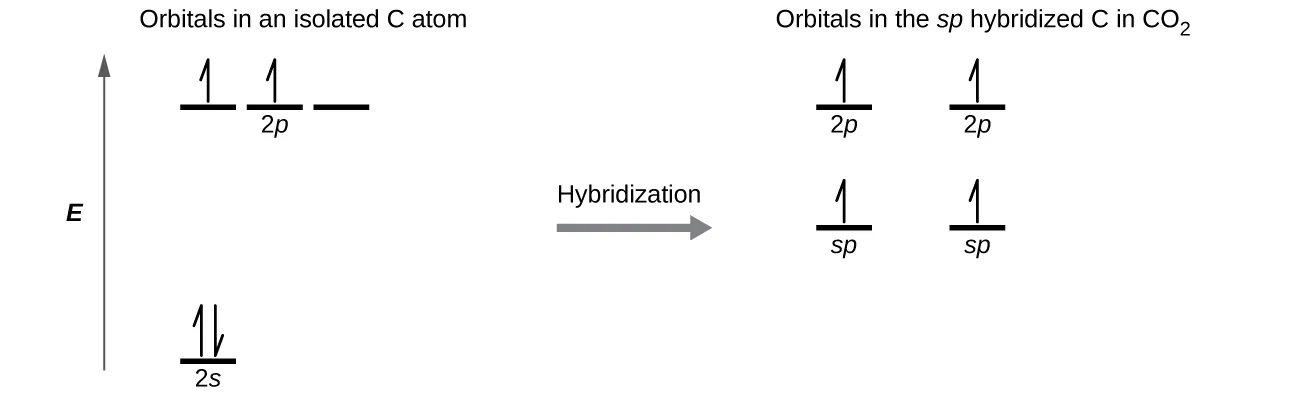Each of the four electrons is in a separate orbital and overlaps with an electron on an oxygen atom.

33.

(a) Similarities: Both are bonding orbitals that can contain a maximum of two electrons. Differences: σ orbitals are end-to-end combinations of atomic orbitals, whereas π orbitals are formed by side-by-side overlap of orbitals. (b) Similarities: Both are quantum-mechanical constructs that represent the probability of finding the electron about the atom or the molecule. Differences: ψ for an atomic orbital describes the behavior of only one electron at a time based on the atom. For a molecule, ψ represents a mathematical combination of atomic orbitals. (c) Similarities: Both are orbitals that can contain two electrons. Differences: Bonding orbitals result in holding two or more atoms together. Antibonding orbitals have the effect of destabilizing any bonding that has occurred.

35.

An odd number of electrons can never be paired, regardless of the arrangement of the molecular orbitals. It will always be paramagnetic.

37.

Bonding orbitals have electron density in close proximity to more than one nucleus. The interaction between the bonding positively charged nuclei and negatively charged electrons stabilizes the system.

39.

The pairing of the two bonding electrons lowers the energy of the system relative to the energy of the nonbonded electrons.

41.

(a) H2 bond order = 1, $H2+H2+$ bond order = 0.5, $H2−H2−$ bond order = 0.5, strongest bond is H2; (b) O2 bond order = 2, $O22+O22+$ bond order = 3; $O22−O22−$ bond order = 1, strongest bond is $O22+;O22+;$ (c) Li2 bond order = 1, $Be2+Be2+$ bond order = 0.5, Be2 bond order = 0, strongest bond is $Li2Li2$;(d) F2 bond order = 1, $F2+F2+$ bond order = 1.5, $F2−F2−$ bond order = 0.5, strongest bond is $F2+;F2+;$ (e) N2 bond order = 3, $N2+N2+$ bond order = 2.5, $N2−N2−$ bond order = 2.5, strongest bond is N2

43.

(a) H2; (b) N2; (c) O; (d) C2; (e) B2

45.

Yes, fluorine is a smaller atom than Li, so atoms in the 2s orbital are closer to the nucleus and more stable.

47.

2+

49.

N2 has s-p mixing, so the π orbitals are the last filled in $N22+.N22+.$ O2 does not have s-p mixing, so the σp orbital fills before the π orbitals.

Order a print copy

As an Amazon Associate we earn from qualifying purchases.